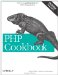# Recipe 7.16. Defining Class Constants

#### 7.16.1. Problem

You want to define constants on a per-class basis, not on a global basis.

#### 7.16.2. Solution

Define them like class properties, but use the const label instead:

`class Math {     const pi = 3.14159; // universal     const  e = 2.71828; // constants } \$area = math::pi * \$radius * \$radius;`

#### 7.16.3. Discussion

PHP reuses its concept of global constants and applies them to classes. Essentially, these are final properties.

Declare them using the const label:

`class Math {     const pi = 3.14159; // universal     const  e = 2.71828; // constants } \$area = math::pi * \$radius * \$radius;`

Like static properties, you can access constants without first instantiating a new instance of your class, and they're accessed using the double colon (::) notation. Prefix the word self:: to the constant name to use it inside of a class.

Unlike properties, constants do not have a dollar sign (\$) before them:

`class Circle {     const pi = 3.14159;     protected \$radius;     public function __construct(\$radius) {         \$this->radius = \$radius;     }     public function circumference() {         return 2 * self::pi * \$this->radius;     } } \$c = new circle(1); print \$c->circumference(); 6.28318`

This example creates a circle with a radius of 1 and then calls the circumference method to calculate its circumference. To use the class's pi constant, refer to it as circumference; otherwise, PHP tries to access circumference value of the global pi constant:

`define('pi', 10); // global pi constant class Circle {     const pi = 3.14159; // class pi constant     protected \$radius;     public function __construct(\$radius) {         \$this->radius = \$radius;     }     public function circumference() {         return 2 * pi * \$this->radius;     } } \$c = new circle(1); print \$c->circumference(); 20`

Oops! PHP has used the value of 10 instead of 3.14159, so the new answer is 20 instead of 6.28318.

Although it's unlikely that you will accidentally redefine Π (you'll probably use the built-in M_PI constant anyway), this can still slip you up.

You cannot assign the value of an expression to a constant, nor can they use information passed into your script:

`// invalid class permissions {     const    read = 1 << 2;     const   write = 1 << 1;     const execute = 1 << 0; } // invalid and insecure class database {     const debug = \$_REQUEST['debug']; }`

Neither the constants in permissions nor the debug constant in database are acceptable because they are not fixed. Even the first example, 1 << 2, where PHP does not need to read in external data, is not allowed.

Since you need to access constants using an explicit name, either self:: or the name of the class, you cannot dynamically calculate the class name during runtime. It must be declared beforehand. For example:

`class Constants {     const pi = 3.14159;     // rest of class here } \$class = 'Constants'; print \$class::pi;`

This produces a parse error, even though this type of construct is legal for non-constant expressions, such as \$class->pi.

Documentation on class constants is available at http://www.php.net/manual/en/language.oop5.constants.php.PHP Cookbook: Solutions and Examples for PHP Programmers
ISBN: 0596101015
EAN: 2147483647
Year: 2006
Pages: 445